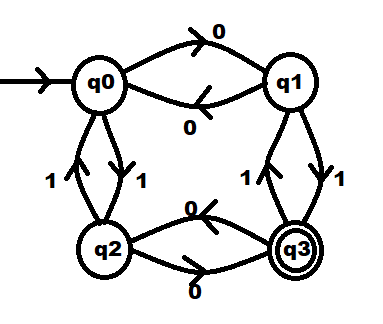Open In App

# Program to construct DFA accepting odd number of 0s and odd number of 1s

Given a binary string S, the task is to write a program for DFA Machine that accepts a string with odd numbers of 0s and 1s.

Examples:

Input: S = “010011”
Output: Accepted
Explanation:
The given string S contains odd number of zeros and ones.

Input: S = “00000”
Output: Not Accepted
Explanation:
The given string S doesn’t contains odd number of zeros and ones.

Approach: Below is the designed DFA Machine for the given problem. Construct a transition table for DFA states and analyze the transitions between each state. Below are the steps:• There are 4 states q0, q1, q2, q3 where q0 is the initial state and q3 is the final state.
• The transition table of the above DFA is as follows:
• Through this table, understand the transitions in the DFA.
• If the final state(q3) is reached after reading the whole string, then the string is accepted otherwise not-accepted.

Below is the implementation of the above approach:

## C++

 `// C++ program for the above approach` `#include ``using` `namespace` `std;` `// Function to check whether the given``// string is accepted by DFA or not``void` `checkValidDFA(string s)``{``    ``// Stores initial state of DFA``    ``int` `initial_state = 0;` `    ``// Stores final state of DFA``    ``int` `final_state;` `    ``// Stores previous state of DFA``    ``int` `previous_state = 0;` `    ``// Iterate through the string``    ``for` `(``int` `i = 0; i < s.length(); i++) {` `        ``// Checking for all combinations``        ``if` `((s[i] == ``'0'``             ``&& previous_state == 0)``            ``|| (s[i] == ``'1'``                ``&& previous_state == 3)) {``            ``final_state = 1;``        ``}``        ``else` `if` `((s[i] == ``'0'``                  ``&& previous_state == 3)``                 ``|| (s[i] == ``'1'``                     ``&& previous_state == 0)) {``            ``final_state = 2;``        ``}``        ``else` `if` `((s[i] == ``'0'``                  ``&& previous_state == 1)``                 ``|| (s[i] == ``'1'``                     ``&& previous_state == 2)) {``            ``final_state = 0;``        ``}``        ``else` `if` `((s[i] == ``'0'``                  ``&& previous_state == 2)``                 ``|| (s[i] == ``'1'``                     ``&& previous_state == 1)) {``            ``final_state = 3;``        ``}` `        ``// Update the previous_state``        ``previous_state = final_state;``    ``}` `    ``// If final state is reached``    ``if` `(final_state == 3) {``        ``cout << ``"Accepted"` `<< endl;``    ``}` `    ``// Otherwise``    ``else` `{``        ``cout << ``"Not Accepted"` `<< endl;``    ``}``}` `// Driver Code``int` `main()``{``    ``// Given string``    ``string s = ``"010011"``;` `    ``// Function Call``    ``checkValidDFA(s);` `    ``return` `0;``}`

## Python3

 `# Python3 program for the above approach` `# Function to check whether the given``# is accepted by DFA or not``def` `checkValidDFA(s):``    ` `    ``# Stores initial state of DFA``    ``initial_state ``=` `0` `    ``# Stores final state of DFA``    ``final_state ``=` `0` `    ``# Stores previous state of DFA``    ``previous_state ``=` `0` `    ``# Iterate through the string``    ``for` `i ``in` `range``(``len``(s)):``        ` `        ``# Checking for all combinations``        ``if` `((s[i] ``=``=` `'0'` `and` `previous_state ``=``=` `0``) ``or``            ``(s[i] ``=``=` `'1'` `and` `previous_state ``=``=` `3``)):``            ``final_state ``=` `1``        ``elif` `((s[i] ``=``=` `'0'` `and` `previous_state ``=``=` `3``) ``or``              ``(s[i] ``=``=` `'1'` `and` `previous_state ``=``=` `0``)):``            ``final_state ``=` `2``        ``elif` `((s[i] ``=``=` `'0'` `and` `previous_state ``=``=` `1``) ``or``              ``(s[i] ``=``=` `'1'` `and` `previous_state ``=``=` `2``)):``            ``final_state ``=` `0``        ``elif` `((s[i] ``=``=` `'0'` `and` `previous_state ``=``=` `2``) ``or``              ``(s[i] ``=``=` `'1'` `and` `previous_state ``=``=` `1``)):``            ``final_state ``=` `3` `        ``# Update the previous_state``        ``previous_state ``=` `final_state` `    ``# If final state is reached``    ``if` `(final_state ``=``=` `3``):``        ``print``(``"Accepted"``)``        ` `    ``# Otherwise``    ``else``:``        ``print``(``"Not Accepted"``)` `# Driver Code``if` `__name__ ``=``=` `'__main__'``:``    ` `    ``# Given string``    ``s ``=` `"010011"` `    ``# Function Call``    ``checkValidDFA(s)` `# This code is contributed by mohit kumar 29`

## Java

 `// Java program for the above approach``import` `java.util.*;` `class` `GFG{``  ` `// Function to check whether the given``// string is accepted by DFA or not``static` `void` `checkValidDFA(String s)``{``    ` `    ``// Stores initial state of DFA``    ``int` `initial_state = ``0``;` `    ``// Stores final state of DFA``    ``int` `final_state = ``0``;` `    ``// Stores previous state of DFA``    ``int` `previous_state = ``0``;` `    ``// Iterate through the string``    ``for``(``int` `i = ``0``; i < s.length(); i++)``    ``{``        ` `        ``// Checking for all combinations``        ``if` `((s.charAt(i) == ``'0'` `&& previous_state == ``0``) ||``            ``(s.charAt(i) == ``'1'` `&& previous_state == ``3``))``        ``{``            ``final_state = ``1``;``        ``}``        ``else` `if` `((s.charAt(i) == ``'0'` `&& previous_state == ``3``) ||``                 ``(s.charAt(i) == ``'1'` `&& previous_state == ``0``))``        ``{``            ``final_state = ``2``;``        ``}``        ``else` `if` `((s.charAt(i) == ``'0'` `&& previous_state == ``1``) ||``                 ``(s.charAt(i) == ``'1'` `&& previous_state == ``2``))``        ``{``            ``final_state = ``0``;``        ``}``        ``else` `if` `((s.charAt(i) == ``'0'` `&& previous_state == ``2``) ||``                 ``(s.charAt(i) == ``'1'` `&& previous_state == ``1``))``        ``{``            ``final_state = ``3``;``        ``}` `        ``// Update the previous_state``        ``previous_state = final_state;``    ``}` `    ``// If final state is reached``    ``if` `(final_state == ``3``)``    ``{``        ``System.out.println(``"Accepted"``);``    ``}` `    ``// Otherwise``    ``else``    ``{``        ``System.out.println(``"Not Accepted"``);``    ``}``}` `// Driver Code``public` `static` `void` `main(String args[])``{``    ` `    ``// Given string``    ``String s = ``"010011"``;` `    ``// Function Call``    ``checkValidDFA(s);``}``}` `// This code is contributed by bgangwar59`

## C#

 `// C# program for the above approach``using` `System;`` ` `class` `GFG{``    ` `// Function to check whether the given``// string is accepted by DFA or not``static` `void` `checkValidDFA(``string` `s)``{``    ` `    ``// Stores initial state of DFA``    ``//int initial_state = 0;`` ` `    ``// Stores final state of DFA``    ``int` `final_state = 0;`` ` `    ``// Stores previous state of DFA``    ``int` `previous_state = 0;`` ` `    ``// Iterate through the string``    ``for``(``int` `i = 0; i < s.Length; i++)``    ``{``        ` `        ``// Checking for all combinations``        ``if` `((s[i] == ``'0'` `&& previous_state == 0) ||``            ``(s[i] == ``'1'` `&& previous_state == 3))``        ``{``            ``final_state = 1;``        ``}``        ``else` `if` `((s[i] == ``'0'` `&& previous_state == 3) ||``                 ``(s[i] == ``'1'` `&& previous_state == 0))``        ``{``            ``final_state = 2;``        ``}``        ``else` `if` `((s[i] == ``'0'` `&& previous_state == 1) ||``                 ``(s[i] == ``'1'` `&& previous_state == 2))``        ``{``            ``final_state = 0;``        ``}``        ``else` `if` `((s[i] == ``'0'` `&& previous_state == 2) ||``                 ``(s[i] == ``'1'` `&& previous_state == 1))``        ``{``            ``final_state = 3;``        ``}`` ` `        ``// Update the previous_state``        ``previous_state = final_state;``    ``}`` ` `    ``// If final state is reached``    ``if` `(final_state == 3)``    ``{``        ``Console.WriteLine(``"Accepted"``);``    ``}`` ` `    ``// Otherwise``    ``else``    ``{``        ``Console.WriteLine(``"Not Accepted"``);``    ``}``}`` ` `// Driver Code``public` `static` `void` `Main()``{``    ` `    ``// Given string``    ``string` `s = ``"010011"``;`` ` `    ``// Function Call``    ``checkValidDFA(s);``}``}` `// This code is contributed by sanjoy_62`

## Javascript

 ``

Output:

`Accepted`

Time Complexity: O(N)
Auxiliary Space: O(1)